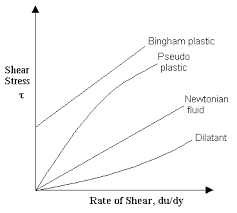# How to Calculate and Solve for Viscosity of Newtonian Fluids | RheologyThe image above represents viscosity of newtonian fluids.

To compute for viscosity of newtonian fluids, two essential parameters are needed and these parameters are Shear Stress (ζ) and Shear Rate (γ).

The formula for calculating viscosity of newtonian fluids:

η = ζ / γ

Where:

η = Viscosity of Newtonian Fluids
ζ = Shear Stress
γ = Shear Rate

Let’s solve an example;
Find the viscosity of newtonian fluids when the shear stress is 15 and the shear rate is 5.

This implies that;

ζ = Shear Stress = 15
γ = Shear Rate = 5

η = ζ / γ
η = 15 / 5
η = 3

Therefore, the viscosity of newtonian fluids is 3.

Calculating for Shear Stress when the Newtonian Fluids and the Shear Rate is Given.

ζ = η x γ

Where;

ζ = Shear Stress
η = Viscosity of Newtonian Fluids
γ = Shear Rate

Let’s solve an example;
Find the shear stress when the newtonian fluids is 20 and the shear rate is 4.

This implies that;

η = Viscosity of Newtonian Fluids = 20
γ = Shear Rate = 4

ζ = η x γ
ζ = 20 x 4
ζ = 80

Therefore, the shear stress is 80.

Calculating for Shear Rate when the Newtonian Fluids and the Shear Stress is Given.

γ = ζ / η

Where;

γ = Shear Rate
η = Viscosity of Newtonian Fluids
ζ = Shear Stress

Let’s solve an example;
Find the shear rate when the newtonian fluids is 10 and the shear stress is 30.

This implies that;

η = Viscosity of Newtonian Fluids = 10
ζ = Shear Stress = 30

γ = ζ / η
γ = 30 / 10
γ = 3

Therefore, the shear rate is 3.

Nickzom Calculator – The Calculator Encyclopedia is capable of calculating the viscosity of newtonian fluids.

To get the answer and workings of the viscosity of newtonian fluids using the Nickzom Calculator – The Calculator Encyclopedia. First, you need to obtain the app.

You can get this app via any of these means:

To get access to the professional version via web, you need to register and subscribe for NGN 2,000 per annum to have utter access to all functionalities.
You can also try the demo version via https://www.nickzom.org/calculator

Android (Paid) – https://play.google.com/store/apps/details?id=org.nickzom.nickzomcalculator
Android (Free) – https://play.google.com/store/apps/details?id=com.nickzom.nickzomcalculator
Apple (Paid) – https://itunes.apple.com/us/app/nickzom-calculator/id1331162702?mt=8
Once, you have obtained the calculator encyclopedia app, proceed to the Calculator Map, then click on Materials and Metallurgical under Engineering.Now, Click on Rheology under Materials and MetallurgicalNow, Click on Viscosity of Newtonian Fluids under RheologyThe screenshot below displays the page or activity to enter your values, to get the answer for the viscosity of newtonian fluids according to the respective parameters which is the Shear Stress (ζ) and Shear Rate (γ).Now, enter the values appropriately and accordingly for the parameters as required by the Shear Stress (ζ) is 15 and Shear Rate (γ) is 5.Finally, Click on CalculateAs you can see from the screenshot above, Nickzom Calculator– The Calculator Encyclopedia solves for the viscosity of newtonian fluids and presents the formula, workings and steps too.